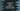# JavaScript program to check if a number is multiple of 3 without using modulo## Introduction :

In this post, I will show you three different ways to check if a number is divisible by 3 or not without using the modulo % operator. For each example, we will use one for loop to iterate from 0 to 30 and print out the numbers those are divisible by 3. It will be a good way to test the methods.

## Method 1:

This is a simple process to check if a number is divisible by 3 or not : Divide the number by 3, convert its value to integer, multiply this result by 3 and check if it is equal to the original number or not.

### JavaScript Program :

``````const isMultipleOfThree = num => {
const div = parseInt(num / 3);

return num === div * 3;
};

for (let i = 0; i <= 30; i++) {
if (isMultipleOfThree(i)) {
console.log(i);
}
}``````

### Explanation :

In this example, we are using one for loop to check for all numbers starting from 0 to 30. The parseInt method is used to get the integer value of the division num/3. It returns true or false based on the original number is equal to divided value * 3 or not.

### Output :

``````0
3
6
9
12
15
18
21
24
27
30``````

## Method 2 : Recursive way :

This is a recursive way to check if a number is divisible by 3 or not. Basically, we will keep subtracting 3 from the original number until it becomes zero or less than zero. If it becomes zero, that number is divisible by 3. And if it is less than zero, it is not.

### JavaScript Program :

``````const isMultipleOfThree = num => {
if (num === 0) return true;

if (num < 0) return false;

return isMultipleOfThree(num - 3);
};

for (let i = 0; i <= 30; i++) {
if (isMultipleOfThree(i)) {
console.log(i);
}
}``````

### Explanation :

Here, isMultipleOfThree is a recursive method. We are calling the method recursively by subtracting 3 each time. If the value is zero, it returns true. Else, it returns false.

### Output :

Similar to the above program, we are running one for loop to check for each numbers starting from 0 to 30. It prints the below output :

``````0
3
6
9
12
15
18
21
24
27
30``````

## Method 3: Find using sum of digits :

One number is divisible by 3 if the sum of its digits is divisible by 3. For example, the sum of digits for 1236 is 1 + 2 + 3 + 6 = 12 = 1 + 2 = 3, which is divisible by 3. So, 1236 is also divisible by 3.

We will write one function to find out the sum of all digits. The main function will call this function. If the sum is 3, 6 or 9, it is divisible by 3.

### JavaScript Program :

``````const sumDigits = num => {
let sum = 0;
while (num) {
sum += num % 10;
num = Math.floor(num / 10);
}
return sum;
};

const isMultipleOfThree = num => {
let sumOfDigits = sumDigits(num);
return (
sumOfDigits === 0 ||
sumOfDigits === 3 ||
sumOfDigits === 6 ||
sumOfDigits === 9
);
};

for (let i = 0; i <= 30; i++) {
if (isMultipleOfThree(i)) {
console.log(i);
}
}``````

Here, isMultipleOfThree checks if a number is divisible by 3 or not. It calls sumDigits to find the sum of all digits. If it is equal to 0, 3, 6 or 9, it returns true. Else, it returns false.

### Sample Output :

It will print the below output :

``````0
3
6
9
12
15
18
21
24
27
30``````